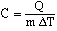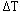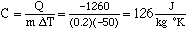Subject: Kelvin scale math problem Name: IRENA Who is asking: Student Level: Secondary Question: hello, my name is irena and i have a question. It's about the Kelvin scale. I know this is a science type of question, but it is also math and all the other science sites never replied to my question. Here's my problem: In transferring 1260J of heat to water, the temperature of 0.20 kg of mercury falls 50 K. Calculate the specific heat capacity of mercury using the equation C= Q/(m)(delta t) i know that Q= -1260J because it's loosing heat and m= 0.2kg and since i have to use 'C i have to convert Kelvins into Celsius (i also know that 'C has to be negative since it looses heat) One Kelvin equals one Celsius degree (they have same intervals), right? so shouldn't mercury falling 50 K = mercury falling 50 'C ? In the question we don't know at what temperature the mercury started at. And we don't know what temperature it ended at either. We can say that mercury started at 350 K and when it was mixed with water it fell 50 K and ended at 300 K. Which would be the same as it starting at 78'C and falling 50 'C to 28'C. This is just an example to clear my point. Please write back telling me if i'm wrong or right and if I am wrong could you please explain because i might have trouble with this later on. Thank-you for your time, irena Hi Irena, In the expressiondesignates CHANGE in temperature. Specific heat depends on the temperature change and not the individual temperatures. (From what I have read this is not exactly true but specific heat's dependence on the temperature is very small and in many cases is ignored.) Thus, using your numbersCheers, Harley Go to Math Central